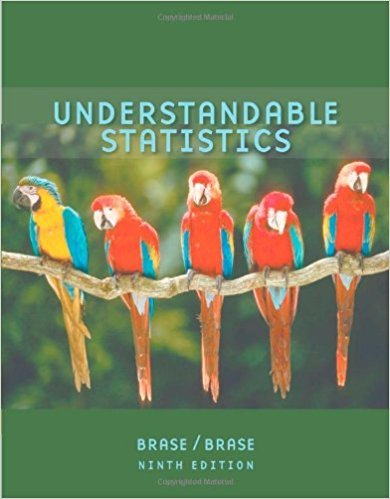×
×

# Solutions for Chapter 8.3: ESTIMATION## Full solutions for Understandable Statistics | 9th Edition

ISBN: 9780618949922Solutions for Chapter 8.3: ESTIMATION

Solutions for Chapter 8.3
4 5 0 383 Reviews
12
5
##### ISBN: 9780618949922

This expansive textbook survival guide covers the following chapters and their solutions. This textbook survival guide was created for the textbook: Understandable Statistics, edition: 9. Since 27 problems in chapter 8.3: ESTIMATION have been answered, more than 38480 students have viewed full step-by-step solutions from this chapter. Chapter 8.3: ESTIMATION includes 27 full step-by-step solutions. Understandable Statistics was written by and is associated to the ISBN: 9780618949922.

Key Statistics Terms and definitions covered in this textbook
• Alias

In a fractional factorial experiment when certain factor effects cannot be estimated uniquely, they are said to be aliased.

• Attribute control chart

Any control chart for a discrete random variable. See Variables control chart.

• Bernoulli trials

Sequences of independent trials with only two outcomes, generally called “success” and “failure,” in which the probability of success remains constant.

• Central limit theorem

The simplest form of the central limit theorem states that the sum of n independently distributed random variables will tend to be normally distributed as n becomes large. It is a necessary and suficient condition that none of the variances of the individual random variables are large in comparison to their sum. There are more general forms of the central theorem that allow ininite variances and correlated random variables, and there is a multivariate version of the theorem.

• Central tendency

The tendency of data to cluster around some value. Central tendency is usually expressed by a measure of location such as the mean, median, or mode.

• Chi-square (or chi-squared) random variable

A continuous random variable that results from the sum of squares of independent standard normal random variables. It is a special case of a gamma random variable.

• Conditional mean

The mean of the conditional probability distribution of a random variable.

• Conidence interval

If it is possible to write a probability statement of the form PL U ( ) ? ? ? ? = ?1 where L and U are functions of only the sample data and ? is a parameter, then the interval between L and U is called a conidence interval (or a 100 1( )% ? ? conidence interval). The interpretation is that a statement that the parameter ? lies in this interval will be true 100 1( )% ? ? of the times that such a statement is made

• Conidence level

Another term for the conidence coeficient.

• Convolution

A method to derive the probability density function of the sum of two independent random variables from an integral (or sum) of probability density (or mass) functions.

• Defect concentration diagram

A quality tool that graphically shows the location of defects on a part or in a process.

• Deming’s 14 points.

A management philosophy promoted by W. Edwards Deming that emphasizes the importance of change and quality

• Design matrix

A matrix that provides the tests that are to be conducted in an experiment.

• Designed experiment

An experiment in which the tests are planned in advance and the plans usually incorporate statistical models. See Experiment

• Discrete uniform random variable

A discrete random variable with a inite range and constant probability mass function.

• Distribution free method(s)

Any method of inference (hypothesis testing or conidence interval construction) that does not depend on the form of the underlying distribution of the observations. Sometimes called nonparametric method(s).

• Estimate (or point estimate)

The numerical value of a point estimator.

• Expected value

The expected value of a random variable X is its long-term average or mean value. In the continuous case, the expected value of X is E X xf x dx ( ) = ?? ( ) ? ? where f ( ) x is the density function of the random variable X.

• Exponential random variable

A series of tests in which changes are made to the system under study

• Forward selection

A method of variable selection in regression, where variables are inserted one at a time into the model until no other variables that contribute signiicantly to the model can be found.

×